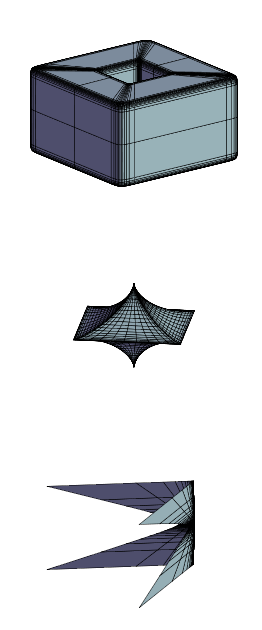In mathematics, the superquadrics or super-quadrics (also superquadratics) are a family of geometric shapes defined by formulas that resemble those of ellipsoids and other quadrics, except that the squaring operations are replaced by arbitrary powers. They can be seen as the three-dimensional relatives of the Lamé curves ("Superellipses").

The superquadrics include many shapes that resemble cubes, octahedra, cylinders, lozenges and spindles, with rounded or sharp corners. Because of their flexibility and relative simplicity, they are popular geometric modeling tools, especially in computer graphics.

Some authors, such as Alan Barr, define "superquadrics" as including both the superellipsoids and the supertoroids. However, the (proper) supertoroids are not superquadrics as defined above; and, while some superquadrics are superellipsoids, neither family is contained in the other.

## Formulas

### Implicit equation

The basic superquadric has the formula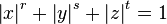$\left|x\right|^r + \left|y\right|^s + \left|z\right|^t =1$

where r, s, and t are positive real numbers that determine the main features of the superquadric. Namely:

• less than 1: a pointy octahedron with concave faces and sharp edges.
• exactly 1: a regular octahedron.
• between 1 and 2: an octahedron with convex faces, blunt edges and blunt corners.
• exactly 2: a sphere
• greater than 2: a cube with rounded edges and corners.
• infinite (in the limit): a cube

Each exponent can be varied independently to obtain combined shapes. For example, if r=s=2, and t=4, one obtains a solid of revolution which resembles an ellipsoid with round cross-section but flattened ends. This formula is a special case of the superellipsoid's formula if (and only if) r = s.

If any exponent is allowed to be negative, the shape extends to infinity. Such shapes are sometimes called super-hyperboloids.

The basic shape above spans from -1 to +1 along each coordinate axis. The general superquadric is the result of scaling this basic shape by different amounts A, B, C along each axis. Its general equation is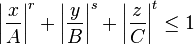$\left|\frac{x}{A}\right|^r + \left|\frac{y}{B}\right|^s + \left|\frac{z}{C}\right|^t \leq 1$

### Parametric description

Parametric equations in terms of surface parameters u and v (longitude and latitude) are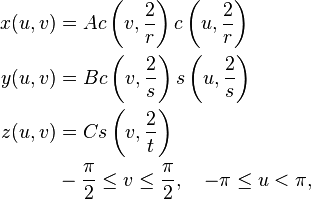\begin{align} x(u,v) &{}= A c\left(v,\frac{2}{r}\right) c\left(u,\frac{2}{r}\right) \\ y(u,v) &{}= B c\left(v,\frac{2}{s}\right) s\left(u,\frac{2}{s}\right) \\ z(u,v) &{}= C s\left(v,\frac{2}{t}\right) \\ & -\frac{\pi}{2} \le v \le \frac{\pi}{2}, \quad -\pi \le u < \pi , \end{align}

where the auxiliary functions are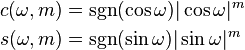\begin{align} c(\omega,m) &{}= \sgn(\cos \omega) |\cos \omega|^m \\ s(\omega,m) &{}= \sgn(\sin \omega) |\sin \omega|^m \end{align}

and the sign function sgn(x) is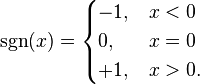$\sgn(x) = \begin{cases} -1, & x < 0 \\ 0, & x = 0 \\ +1, & x > 0 . \end{cases}$

## Plotting code

The following GNU Octave code generates a mesh approximation of a superquadric:

 function retval=superquadric(epsilon,a)
n=50;
etamax=pi/2;
etamin=-pi/2;
wmax=pi;
wmin=-pi;
deta=(etamax-etamin)/n;
dw=(wmax-wmin)/n;
[i,j] = meshgrid(1:n+1,1:n+1)
eta = etamin + (i-1) * deta;
w   = wmin + (j-1) * dw;
x = a(1) .* sign(cos(eta)) .* abs(cos(eta)).^epsilon(1) .* sign(cos(w)) .* abs(cos(w)).^epsilon(1);
y = a(2) .* sign(cos(eta)) .* abs(cos(eta)).^epsilon(2) .* sign(sin(w)) .* abs(sin(w)).^epsilon(2);
z = a(3) .* sign(sin(eta)) .* abs(sin(eta)).^epsilon(3);

mesh(x,y,z);
endfunction;


## References

1. Barr, A.H. (January 1981), Superquadrics and Angle-Preserving Transformations. IEEE_CGA vol. 1 no. 1, pp. 11–23
2. Barr, A.H. (1992), Rigid Physically Based Superquadrics. Chapter III.8 of Graphics Gems III, edited by D. Kirk, pp. 137–159
• Jaklič, A., Leonardis, A., Solina, F., Segmentation and Recovery of Superquadrics. Kluwer Academic Publishers, Dordrecht, 2000.